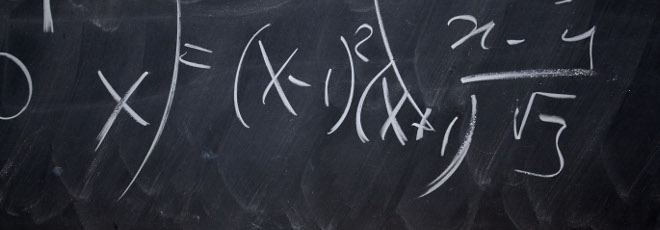## “Strong Sard Conjecture in three dimensional analytic manifolds”

Canceled Martedì 5 Aprile 2022, ore 14:30 - Aula 1BC45 e Zoom - André Belotto (IMJ-PRG, Université de Paris)

Abstract

Given a totally nonholonomic distribution of rank two $\Delta$ on a three-dimensional manifold $M$, it is natural to investigate the size of the set of points $\mathcal{X}^x$ that can be reached by singular horizontal paths starting from a same point $x \in M$. In this setting, the Sard conjecture states that $\mathcal{X}^x$ should be a subset of the so-called Martinet surface of 2-dimensional Hausdorff measure zero.

I will present a reformulation of the conjecture in terms of the behavior of a (real) singular foliation. Next, I will present a recent work in collaboration with A. Figalli, L. Rifford and A. Parusinski, where we show that the (strong version of the) conjecture holds in the analytic category and in dimension 3. Our methods rely on resolution of singularities of surfaces, foliations and metrics; regularity analysis of Poincaré transition maps; and on a symplectic argument, concerning a transversal metric of an isotropic singular foliation.